# Square metal sheet

Four squares of 300 mm side were cut out from a square sheet metal plate with a side of 0,7 m. Express the fraction and the percentage of waste from the square metal sheet.

Result

f =  0.265
p =  26.5 %

#### Solution:

$a = 0.7 \ m \ \\ b = 300 \ mm = 300 / 1000 \ m = 0.3 \ m \ \\ \ \\ S_{ 1 } = a^2 = 0.7^2 = \dfrac{ 49 }{ 100 } = 0.49 \ m^2 \ \\ S_{ 2 } = 4 \cdot \ b^2 = 4 \cdot \ 0.3^2 = \dfrac{ 9 }{ 25 } = 0.36 \ \\ \ \\ o = S_{ 1 }-S_{ 2 } = 0.49-0.36 = \dfrac{ 13 }{ 100 } = 0.13 \ m^2 \ \\ f = \dfrac{ o }{ S_{ 1 } } = \dfrac{ 0.13 }{ 0.49 } = \dfrac{ 13 }{ 49 } \doteq 0.2653 = 0.265$
$p = 100 \cdot \ f = 100 \cdot \ 0.2653 = \dfrac{ 53 }{ 2 } = 26.5 = 26.5 \%$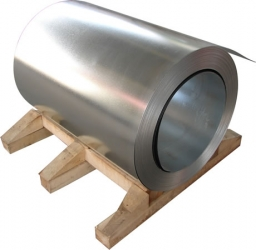Our examples were largely sent or created by pupils and students themselves. Therefore, we would be pleased if you could send us any errors you found, spelling mistakes, or rephasing the example. Thank you!

Leave us a comment of this math problem and its solution (i.e. if it is still somewhat unclear...):Be the first to comment!Tips to related online calculators
Need help calculate sum, simplify or multiply fractions? Try our fraction calculator.

## Next similar math problems:

1. SquaresFrom a square of 12 dm perimeter is cut smaller square, whose side is the third side of the square. What is the area of the rest?
2. Tripled squareIf you tripled the length of the sides of the square ABCD you increases its content by 200 cm2. How long is the side of the square ABCD?
3. Star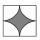Calculate the content of the shaded part of square with side 28 cm.
4. 6 regular polygonIt is given 6 side regular polygon whose side is 5 cm. Calculate its content area. Compare how many more cm2 (square centimeters) has a circle in which is inscribed the 6-gon.
5. WasteHow many percents are waste from a circular plate with a radius of 1 m from which we cut a square with the highest area?
6. Square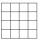Square JKLM has sides of length 24 cm. Point S is the center of LM. Calculate the area of the quadrant JKSM in cm2.
7. Company logoThe company logo consists of a blue circle with a radius of 4 cm, which is an inscribed white square. What is the area of the blue part of the logo?
8. Two gardenersThe garden with an area of 81 square meters was divided by two gardeners in a ratio of 4: 5. How much did the second gardener get more first?
9. Circular ringSquare with area 16 centimeters square are inscribed circle k1 and described circle k2. Calculate the area of circular ring, which circles k1, k2 form.
10. Garden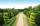The garden has a rectangular shape with lengths of 25 and 40 meters. It has been expanded so that each of its size increased by one fifth. How many square meters increased its acreage?
11. Desribed circle to rectangle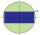Rectangle with sides 6 cm and 4 cm was circumscribed circle. What part of the content of the circle determined by the circumscribed circle occupies a rectangle? Express in perctentages(%).
12. Octagon from rectangleFrom tablecloth rectangular shape with dimensions of 4 dm and 8 dm we cuts down the corners in the shape of isosceles triangles. It thus formed an octagon with area 26 dm2. How many dm2 we cuts down?
13. Decorative fenceThe rectangular garden is 57 m long and 42 m wide. Calculate how much m² it will reduce if it encloses with a 60 cm wide decorative fence.
14. Rectangle 39Find the perimeter and area of the rectangular with vertices (-1, 4), (0,4), (0, -1), and (-4, 4)
15. The terrace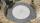Around the round pool with a diameter of 5.5 meters is a wooden terrace with a width of 130 cm. What is the area of the terrace?
16. Annulus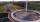Calculate the area of two circles annulus k1 (S, 3 cm) and k2 (S, 5 cm).
17. Annulus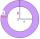Two concentric circles form an annulus of width 10 cm. The radius of the smaller circle is 20 cm. Calculate the content area of annulus.